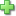# Basic Math Skills 4

Current Retail Price: \$29.99
Not in stock

See series description for full review.

In Basic Math Skills, Grade 4 you’ll find just what you need to provide extra skill practice for individual students, small groups, or the whole class. These reproducible pages are perfect for homework too.

Skills practiced include:

Number and Operations

• Read, write, compare, use place value and round numbers
• Add, subtract, multiply, and divide whole numbers
• Identify odds, evens, primes, composites, factors, and multiples
• Identify, compare, add and subtract fractions
• Determine common equivalent fractions and decimals
• Compare sets and values using <, >, and =

Algebra

• Describe and extend visual and numerical patterns
• Identify number relationships (fact families), utilizing properties
• Use function table (input/output tables)
• Locate and plot ordered pairs on a coordinate graph

Geometry

• Identify characteristics of lines, line segments, points, rays, and angles
• Draw, compare, and classify two- and three-dimensional figures
• Identify congruent shapes using rotations, translations, and reflections
• Identify lines of symmetry in two-dimensional shapes

Measurement

• Count mixed coins and compute change
• Identify and order metric
• Compare, use, and convert customary and metric units of linear measurement
• Identify and compare customary and metric units of capacity
• Read a thermometer and solve problems related to temperature
• Find perimeter and area of simple shapes
• Tell time and calculate elapsed time

Data Analysis and Probability

• Construct and interpret graphs
• Create and critique survey questions
• Analyze data utilizing range, median, and mode
• Collect and record data related to simple chance situations
• Compute theoretical probabilities for simple chance events
• Use counting techniques, tree charts, and organized lists
Did you find this review helpful?
Series Description
Related Categories
Recommended for...
Customer ReviewsClick here to write a review